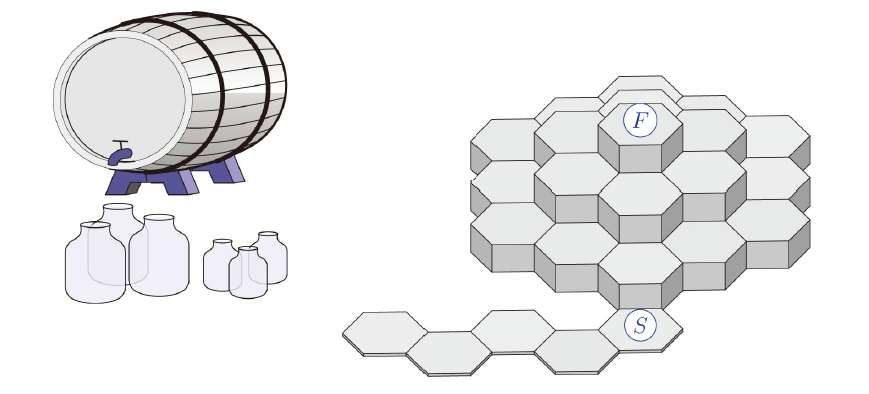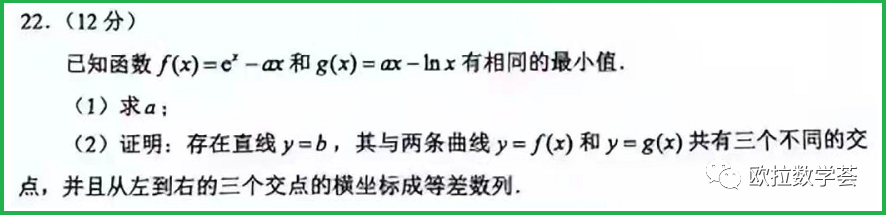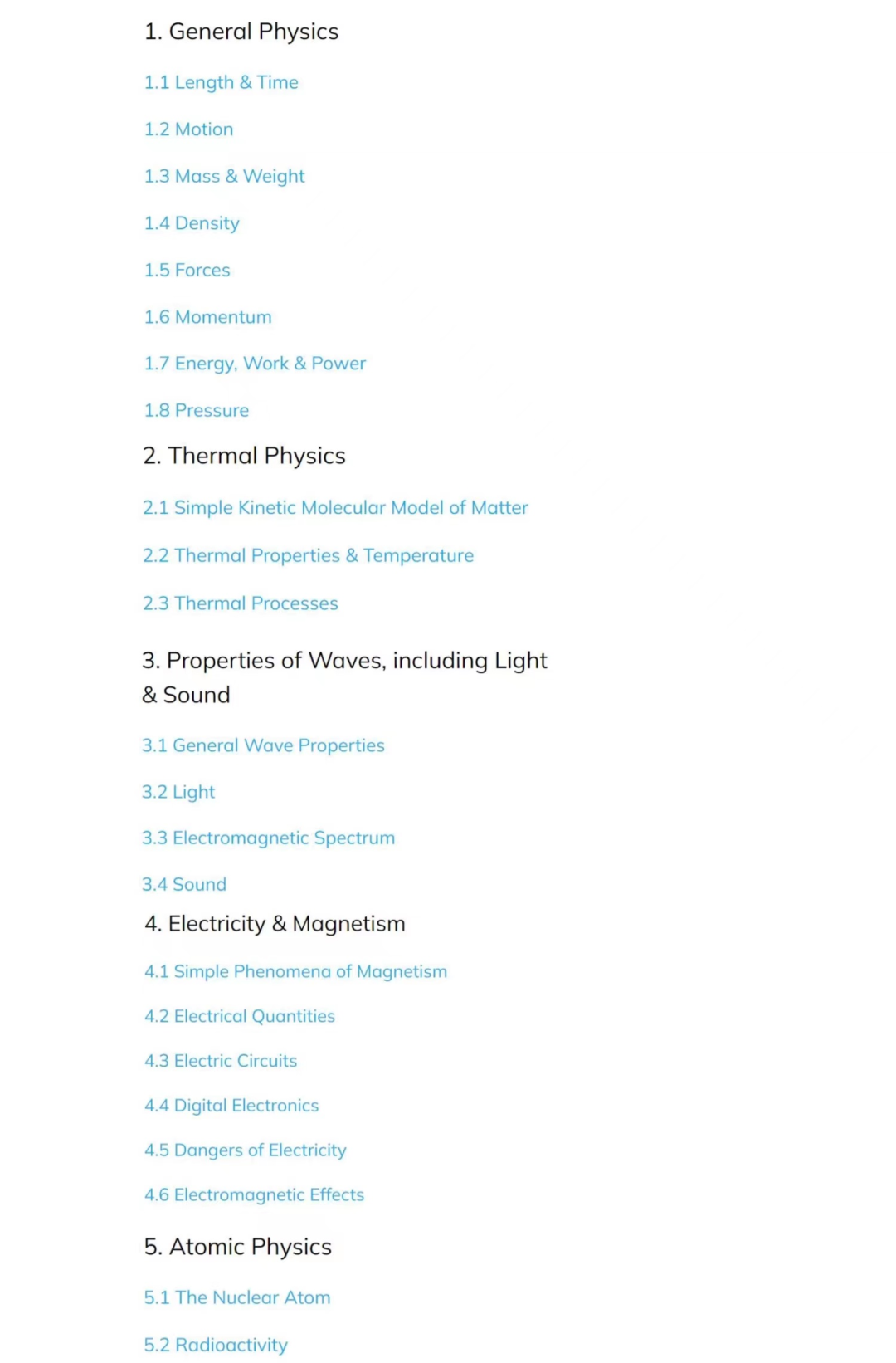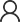# 2022年高考数学压轴题难不难？f'(x)=ex-a,  g'(x)=a-1/x.

f'(x)=0得驻点x=lna，由g'(x)=0得驻点x=1/a

f''(x)=ex,  g''(x)=1/x2.

f(lna)=a(1-lna),  g(1/a)=1+lna.

f(p)=f(q)=g(q)=g(r)=b.### IGCSE阶段物理课程应该怎么学？5. ### 2022年全球顶尖理科国际赛事汇总商务合作在线咨询